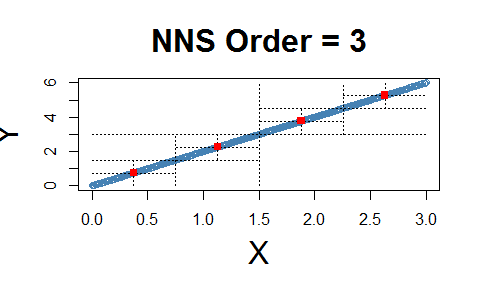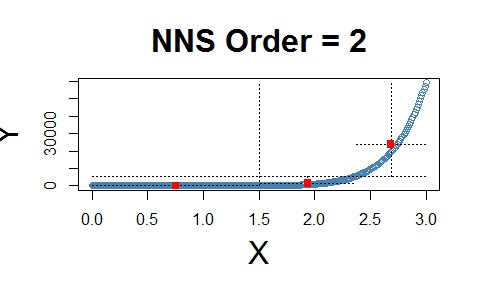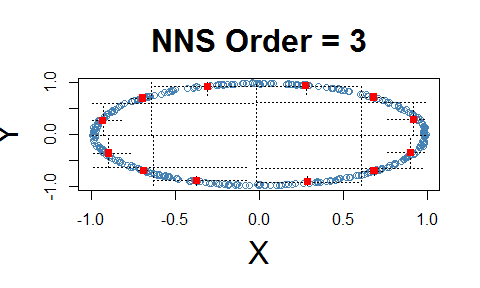# Getting Started with NNS: Correlation and Dependence

require(NNS)
require(knitr)
require(rgl)
require(data.table)

# Correlation and Dependence

The limitations of linear correlation are well known. Often one uses correlation, when dependence is the intended measure for defining the relationship between variables. NNS dependence NNS.dep is a signal:noise measure robust to nonlinear signals.

Below are some examples comparing NNS correlation NNS.cor and NNS.dep with the standard Pearson’s correlation coefficient cor.

## Linear Equivalence

Note the fact that all observations occupy the co-partial moment quadrants.

x = seq(0, 3, .01) ; y = 2 * x

cor(x, y)
##  1
NNS.dep(x, y, print.map = TRUE, order = 3)## $Correlation ##  1 ## ##$Dependence
##  1

## Nonlinear Relationship

Note the fact that all observations occupy the co-partial moment quadrants.

x=seq(0, 3, .01) ; y = x ^ 10

cor(x, y)
##  0.6610183
NNS.dep(x, y, print.map = TRUE, order = 3)## $Correlation ##  0.9413457 ## ##$Dependence
##  0.9413457

## Dependence

Note the fact that all observations occupy only co- or divergent partial moment quadrants for a given subquadrant.

set.seed(123)
df <- data.frame(x = runif(10000, -1, 1), y = runif(10000, -1, 1))
df <- subset(df, (x ^ 2 + y ^ 2 <= 1 & x ^ 2 + y ^ 2 >= 0.95))
NNS.dep(df$x, df$y, print.map = TRUE)## $Correlation ##  -0.007630343 ## ##$Dependence
##  0.9963612